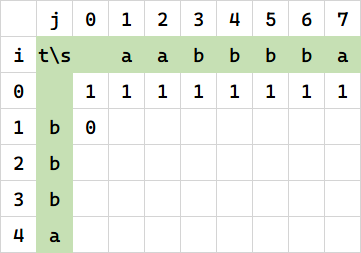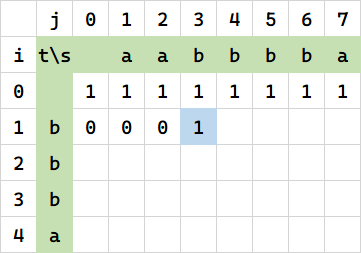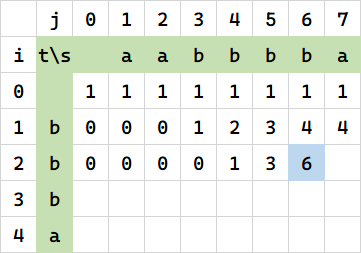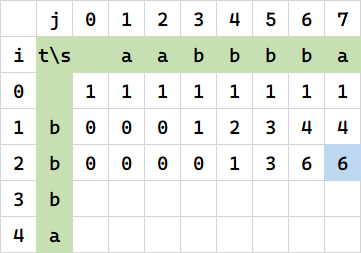# 把“不同的子序列”问题说透彻

## 一个例子• 左边 3，是因为 \$C_3 ^2 = 3\$。

• 左上 3，是因为 \$C_3^1 = 3\$

• 正上 4，是因为 \$C_4^1 = 4\$1. 如果代表串末尾字符不相等，那么就只能删掉这个字符。相当于它左边的格子。

当 s[j] == t[i] 时，\$f(i,j) = f(i, j-1)\$

2. 如果代表串末尾字符相等，那么一方面左边的格子可以继承，另一方面也还要加上左上方的格子。这是组合数的递推公式决定的。

当 s[j] == t[i] 时，\$f(i,j) = f(i-1,j-1)+f(i, j-1)\$

## 组合数递推公式

12 13 14 15, 23 24 25, 34 35, 45

## 回到例子

\$s[0:j]\$ 中包含 \$t[0:i]\$ 的子序列情况，要么涉及了 \$s[j]\$，要么不涉及。

• 如果涉及，数量是 \$f(i,j-1)\$ （即例子中求 `R(aabbbba, bb)`，相当于求 `R(aabbbb, bb)`

• 如果不涉及，则数量是 \$f(i-1,j-1)\$. （即例子中求 `R(aabbbb, b)`